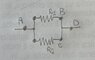# Electric potential difference at the ends of a resistor

• greg_rack

#### greg_rack

Gold Member
So, having two parallel resistor ##R_{1}## and ##R_{2}## , the current flowing through the equivalent one will be ##I_{eq}=I_{1}+I_{2}##.
Now, it comes the point I'm not totally getting: why is ##V_{eq}=V_{1}=V_{2}##? These V's are the difference of potential measured between which points?referring to this ugly image representing the two parallel resistors:
is ##V_{1}=V_{B}-V_{A}## or ##V_{1}=V_{D}-V_{A}##? Or, is ##V_{B}-V_{D}## since they're in the same conductor?
As you can see, I think I haven't clear how does potential varies after that current passes through a resistor.

Look at the circuit. By convention the straight pieces connecting the resistors (wiggly lines) are equipotentials. This means that the potential difference between any two points, which have no circuit element between them, are at the same potential.

•vanhees71
Look at the circuit. By convention the straight pieces connecting the resistors (wiggly lines) are equipotentials. This means that the potential difference between any two points, which have no circuit element between them, are at the same potential.
So, in this case, ##B## and ##C##(?)

So, in this case, ##B## and ##C##(?)
Yes, and also D. You have VAB = VAC = VAD.

•etotheipi and greg_rack
Yes, and also D. You have VAB = VAC = VAD.
Got it, thanks!